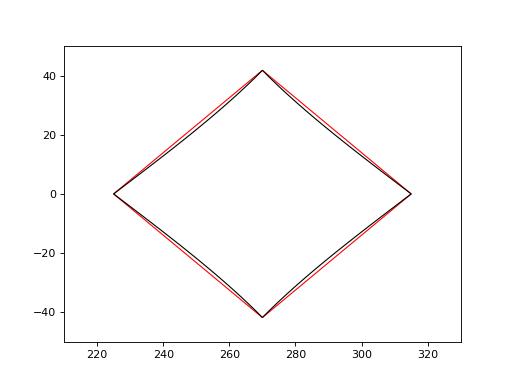# Pixel corners and edges¶

In some cases, you may need to find out the longitude/latitude or celestial coordinates of the corners or edges of HEALPix pixels.

The boundaries_lonlat() method can be used to sample points long the edge of one or more HEALPix pixels:

>>> from astropy_healpix import HEALPix
>>> hp = HEALPix(nside=16, order='nested')
>>> hp.boundaries_lonlat(, step=1)
(<Longitude [[ 1.17809725, 1.08747438, 1.12199738, 1.20830487]] rad>, <Latitude [[ 0.94842784, 0.89458259, 0.84022258, 0.89458259]] rad>)


This method takes a step argument which specifies how many points to sample along each edge. Setting step to 1 returns the corner positions, while setting e.g. 2 returns the corners and points along the middle of each edge, and larger values can be used to get the precise curved edges of the pixels.

The following example shows the difference between the boundary constructed from just the corners (in red) and a much higher-resolution boundary computed with 100 steps on each side (in black):

import numpy as np
from astropy import units as u
import matplotlib.pyplot as plt
from matplotlib.patches import Polygon
from astropy_healpix.core import boundaries_lonlat

ax = plt.subplot(1, 1, 1)

for step, color in [(1, 'red'), (100, 'black')]:
lon, lat = boundaries_lonlat(, nside=1, step=step)
lon = lon.to(u.deg).value
lat = lat.to(u.deg).value
vertices = np.vstack([lon.ravel(), lat.ravel()]).transpose()
p = Polygon(vertices, closed=True, edgecolor=color, facecolor='none')

plt.xlim(210, 330)
plt.ylim(-50, 50)As for other methods, the HEALPix class has an equivalent boundaries_skycoord() method that can return the celestial coordinates of the boundaries as a SkyCoord object if the frame is set:

>>> from astropy.coordinates import Galactic
>>> hp = HEALPix(nside=16, order='nested', frame=Galactic())
>>> hp.boundaries_skycoord(, step=1)
<SkyCoord (Galactic): (l, b) in deg
[[( 67.5       ,  54.3409123 ), ( 62.30769231,  51.25580695),
( 64.28571429,  48.14120779), ( 69.23076923,  51.25580695)]]>